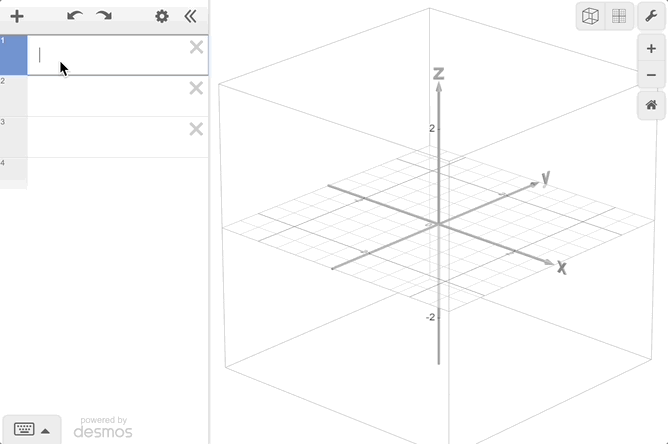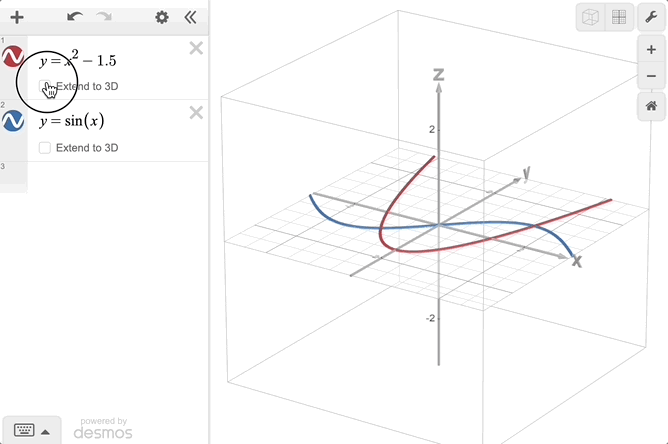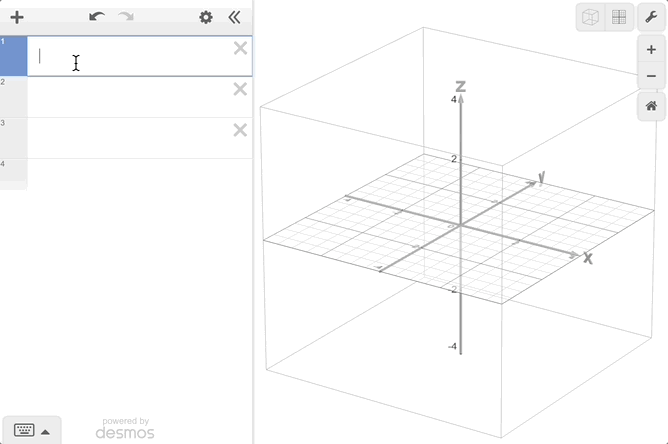# Getting Started: Desmos 3D

Welcome! The Desmos 3D Calculator is a powerful and interactive tool that allows you to jump into a new dimension of math. Desmos 3D is built on the 2D calculator, allowing you to bring your familiarity with the Cartesian plane into a three dimensional space. Get started below or check out the Desmos 3D User Guide.

### Navigating the 3D Cube

When you visit desmos.com/3d, you'll see a place to write expressions on the left and the 3D cube on the right. The $$x$$, $$y$$, and $$z$$ axes sit inside of the 3D cube. By default, the 3D cube is rotated slightly so you can see two of its sides, and the $$XY$$ plane is tilted to face you. Try graphing a point like $$(0,1,2)$$ or a plane like $$z=3$$. You can click and drag to rotate the cube. If you click, drag, and then release the cube while your cursor is still in motion, the graph will continue to rotate. To stop the rotation, click anywhere on the screen. Similar to the 2D calculator, you can add a new item with the plus button in the top left, open the keypad with the keypad button in the bottom left, and adjust the graph settings with the wrench button in the top right.### Visualizing 3D Surfaces

Desmos 3D allows you to extend your intuition from 2D to 3D. Starting with a 2D equation, you can check the ‘Extend to 3D’ box to watch your curve animate into a 3D surface. You can view anything graphed in 3D on the 2D plane by pressing the 'XY Orientation' button in the top right of the screen. Press the 'Default Orientation' button to get back to the 3D cube. Read more about extending from 2D to 3D in our 2D to 3D help center article.There are powerful ways to investigate the intersection of surfaces. One way to look at intersections is by restricting the domain of a surface. For example, restricting $$x^2+y^2$$ to the domain $${z=3}$$ will graph the slice of the surface where $$z=3$$. Another way to visualize this intersection is to check the translucent surfaces box in the graph settings menu in the top right. With this option, you can see through the surfaces of the graph of $$x^2+y^2$$ and the plane $${z=3}$$ to visualize how the two intersect.#### Example Graphs

The example graphs can be accessed by pressing on the hamburger menu in the top left of the screen and scrolling down past your saved graphs. Here, you can get an idea of some of the curves and surfaces you can graph in Desmos 3D. You can open any of the graphs and edit the equations to learn more. The surface of revolution example graph can be used as a template to revolve an equation in terms of $$x$$ around the $$x$$-axis. Example graphs are one great place to start to learn about the power of Desmos 3D.

#### Quests

Another way to get started is by trying a quest built into the 3D Calculator. You can choose a quest from the announcement in the expression list. Each quest is a challenge to complete through a series of goals and tips. Challenge yourself to complete them all or send one to a friend to complete.

#### Next Steps

We can't wait for you to dive into 3D with us. Be sure to check out the 3D User Guide that can be found in the help menu in the calculator. If you want to dive deeper, check out our Spherical Coordinates article. Please write in with any questions or feedback to support@desmos.com.